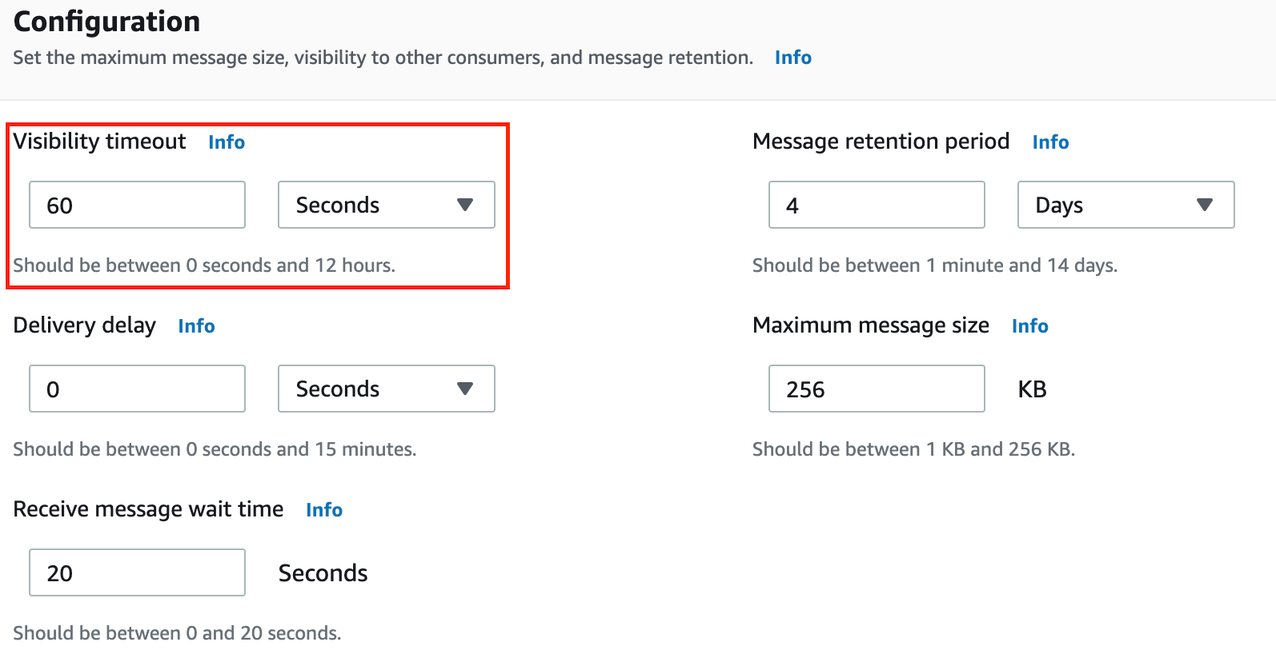# 实时数据处理中的AWS lambda (python) 性能优化

## 二、程序上的调优

### 1. 逻辑的基本优化

（1）首先，考虑把程序初始化从循环体内移到循环体外，示例如下：

``````for key in s3_source:
s3=boto3.resource('s3')
…

s3=boto3.resource('s3')
for key in s3_source:``````

（2）再次，减少不必要的包引用或处理，比如import <library> 等，这常见于经过多次改版的程序。

（3）只选择需要的字段

AWS Lambda里往往会使用到pandas包的dataframe，dataframe中的字段并不一定都是我们所需要的。除了减少数据量，还可以通过减少需要处理的dataframe字段数目来降低dataframe的内存消耗，降低处理时长。

（4）然后，把加工新增的字段放在排序操作之后,比如：

``````df = df.sort_values(['id', 'eventTime'], ignore_index=True)
df['idx'] = df.id  # 新增字段
``````

``````df['idx'] = df.id  # 新增字段
df = df.sort_values(['id', 'eventTime'], ignore_index=True)``````
`这是为了减少待排序的数据量，对于海量数据排序操作会有明显的性能提升。`

（5）使用条件过滤的时候，尽可能把过滤条件在数据处理过程中前移，其基本思想也是为了减少需要处理的数据量。

### 2. 基于不同语言如python对应的优化

（1）使用矢量化计算代替行操作

``````def calculatetime(df):
if (df['starttime_y'] <= df['endtime_y']):
return df['endtime_y']-df['starttime_y']
df[index_name] = df.apply(calculatetime, axis = 1)
``````

``df.loc[(df['starttime_y'] <= df['endtime_y']), index_name] = df['endtime_y'] - df['starttime_y']``

（2）数据类型替换

（3）对象删除及垃圾回收

``````import gc
var1 = ...
var2 = ...
del var1, var2
gc.collect()
gc.garbage
``````

## 三、触发(trigger)方面的改进

（1）SQS Visibility timeout（2）SQS delete message

``````queue_url = 'https://sqs.<region>.amazonaws.com/...'
sqs_client = boto3.client('sqs')
receiptHandle = event["Records"]["receiptHandle"]
sqs_client.delete_message(QueueUrl=queue_url, ReceiptHandle=receiptHandle)
``````

## 四、I/O(Input/Output)的处理

（1）替代pandas的IO

``````# Read S3 parquet files in the specific S3 bucket key
import pyarrow as pa
df = pa.parquet.ParquetDataset(path, filesystem=pa.fs.S3FileSystem())

# Write S3 parquet with data partitioning
table = pa.Table.from_pandas(df)
partition_cols = ['year', 'month', 'day']
pa.dataset.write_dataset(table, path, basename_template=file_name, format="parquet",
partitioning=partition_cols, filesystem=pa.fs.S3FileSystem(),
existing_data_behavior='delete_matching',
partitioning_flavor='hive')

# Read S3 csv files in the specific S3 bucket key
import awswrangler

（2）基于内存处理后再落盘

（3）File compaction

``````df = pq.ParquetDataset(
s3=boto3.resource('s3')
s3_source = s3.Bucket(bucket).objects.filter(Prefix=key)
count_obj = sum(1 for _ in s3_source.all())
if count_obj>1:
for key1 in s3_source:
copy_source = {
'Bucket': bucket,
'Key': key1.key
}
move_after_key = KEY + "pre-compaction/"+"/".join(key1.key.split('/')[1:])
if(len(list(s3.Bucket(bucket).objects.filter(Prefix=move_after_key)))==0):
s3.Bucket(bucket).copy(copy_source, move_after_key)
s3.Object(bucket,key1.key).delete()

iob_df=io.BytesIO()
df.to_parquet(iob_df,index=False)
boto3.client('s3', region_name=REGION).put_object(Bucket=bucket,
Key=key+target_file,Body=iob_df.getvalue())
``````

## 本篇作者### 樊在阔### 孔庆强

AWS专业服务团队的大数据顾问，十多年从事数据湖仓及数据分析，为客户提供数据建模、数据治理及整体专业数据解决方案。在个人爱好方面，喜爱健身、音乐、旅行。### 李烨炜

AWS专业服务团队大数据咨询顾问。专注于企业级客户云上数据架构与数据平台设计等相关咨询服务。

TAGS: